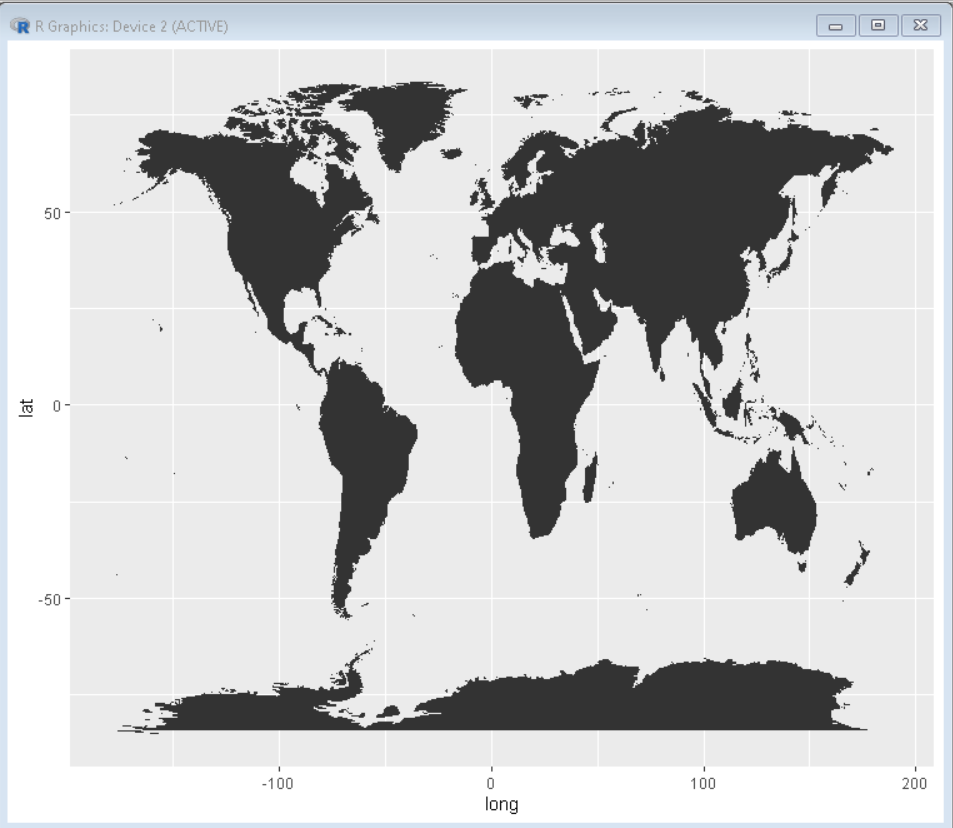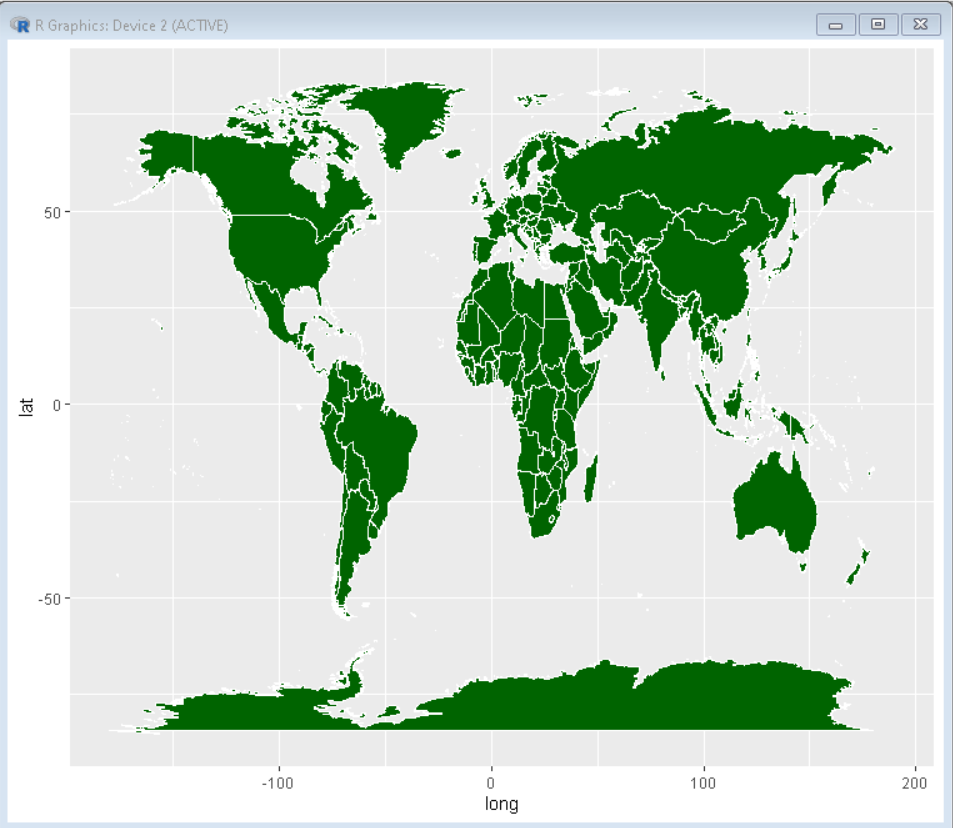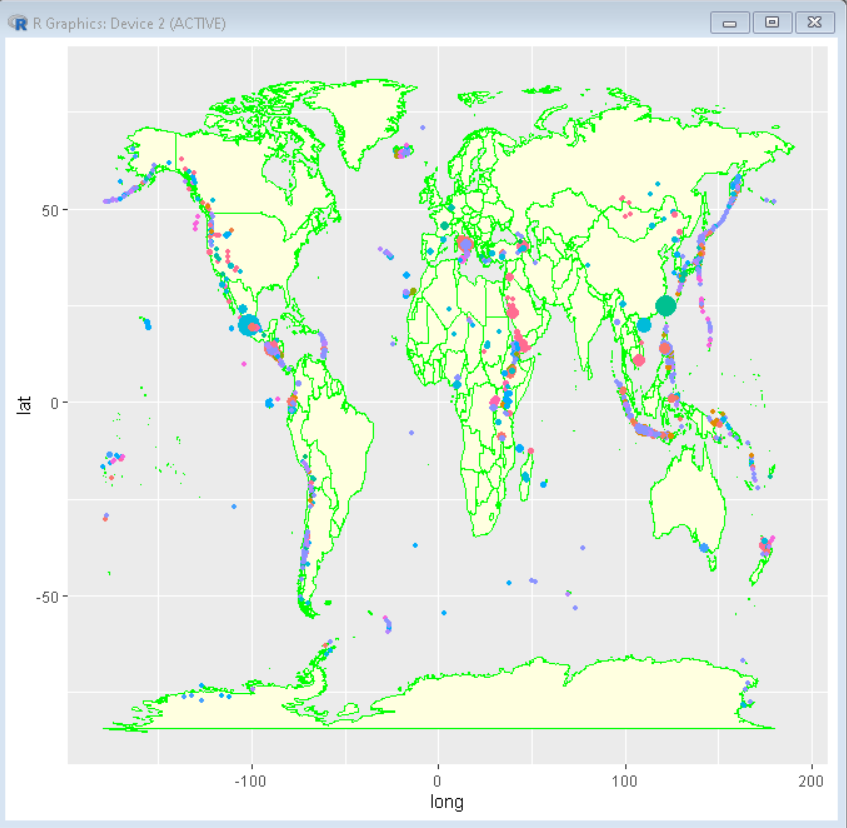Open In App
Related Articles
• Write an Interview Experience
• Data visualization with R and ggplot2

# How To Make World Map with ggplot2 in R?

In this article, we will discuss how to create a world map and plot data on it using the R Programming Language.

To create a world map using it we will use the geom_map() function of the ggplot2 package of the R Language. This function returns a ggplot object so all the functions that work on other ggplot plots will be working in geom_map() too.

Syntax:

ggplot() + geom_map( data, map, aes() )

where,

• data: determines the data be displayed in this layer.
• map: determines the data frame that contains the map coordinates
• aes(): determines the aesthetics that is coordinate system variable and other aesthetical aspects.

Example:

Here, is a basic world map plot made using the geom_map() function. Data frame for map coordinates is taken using the map_data() function. This creates a world map as a ggplot object that can be formatted, modified, and plotted over as a layer just like every other ggplot2 plot.

## R

 `# load library tidyverse``library``(tidyverse)`` ` `# create data for world coordinates using ``# map_data() function``world_coordinates <- ``map_data``(``"world"``)`` ` `# create world map using ggplot() function``ggplot``() +`` ` `# geom_map() function takes world coordinates ``# as input to plot world map``  ``geom_map``(``    ``data = world_coordinates, map = world_coordinates,``    ``aes``(long, lat, map_id = region)``  ``)`

Output:

This is a basic map with default color scheme of ggplot2 package plots.## Color Customization

We can customize the visual look and feel of the plot using the color, fill, and size parameter of the geom_map() function.

Syntax:

ggplot() + geom_map( data, map, aes(), size, color, fill )

where,

• size: determines the thickness of the border of the map
• color: determines the color of the border of the map
• fill: determines the color of the fill background of the map

Example:

Here, is a world map with green fill and thick white border made using the fill, color, and size property of the geom_map() function. This makes the map more visually appealing and helps us differentiate different countries due to the contrast of white boundaries.

## R

 `# load library tidyverse``library``(tidyverse)`` ` `# create data for world coordinates using ``# map_data() function``world_coordinates <- ``map_data``(``"world"``)`` ` `# create world map using ggplot() function``ggplot``() +`` ` `# geom_map() function takes world coordinates as input ``# to plot world map color parameter determines the ``# color of borders in map fill parameter determines ``# the color of fill in map size determines the thickness``# of border in map``  ``geom_map``(``    ``data = world_coordinates, map = world_coordinates,``    ``aes``(long, lat, map_id = region)``    ``color = ``"white"``, fill = ``"darkgreen"``, size = 0.2``  ``)`

Output:## Plotting data over the world map

Since the geom_map() function returns the ggplot() object we can plot data over it just like on any other ggplot plot. To do so we just need latitude and longitude data in our dataset to plot the data on the map. We can plot a line plot, scatter plot, boxplot or any other desired plot over the world map plot using the desired ggplot2 function.

Syntax:

ggplot()+ geom_map()+ geom_plot()

where,

• geom_plot(): determines any plot function from ggplot2 package

Example:

Int his example we have plotted a volcanic eruption dataset on top of geom_map() function using geom_point() function. Here we can show the variation of the intensity of volcanic eruptions around the world. this helps us in understanding the critical zone for volcanic eruption.

Dataset Used: volcano

## R

 `# load library tidyverse``library``(tidyverse)`` ` `# create data for world coordinates using map_data() function``world_coordinates <- ``map_data``(``"world"``)`` ` `# read volcano_eruption data from volcano.csv``volcano_eruption <- readr::``read_csv``(``"volcano.csv"``)`` ` `# create world map using ggplot() function``ggplot``() +``# geom_map() funct``ion takes world coordinates as input``# to plot world map color parameter determines the``# color of borders in map fill parameter determines the``# color of fill in map size determines the thickness of``# border in map``  ``geom_map``(``    ``data = world, map = world,``    ``aes``(long, lat, map_id = region),``    ``color = ``"green"``, fill= ``"lightyellow"``  ``)+``# geom_point function is used to plot scatter plot on top ``# of world map``geom_point``(``    ``data = volcano_eruption,``    ``aes``(longitude, latitude, color = primary_volcano_type,``        ``size=population_within_10_km),``    ``alpha = 1``  ``) +`` ` `# legend.position as none removes the legend``theme``(legend.position=``"none"``)`

Output: